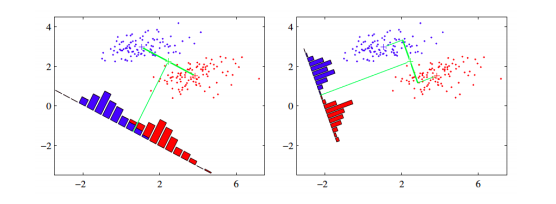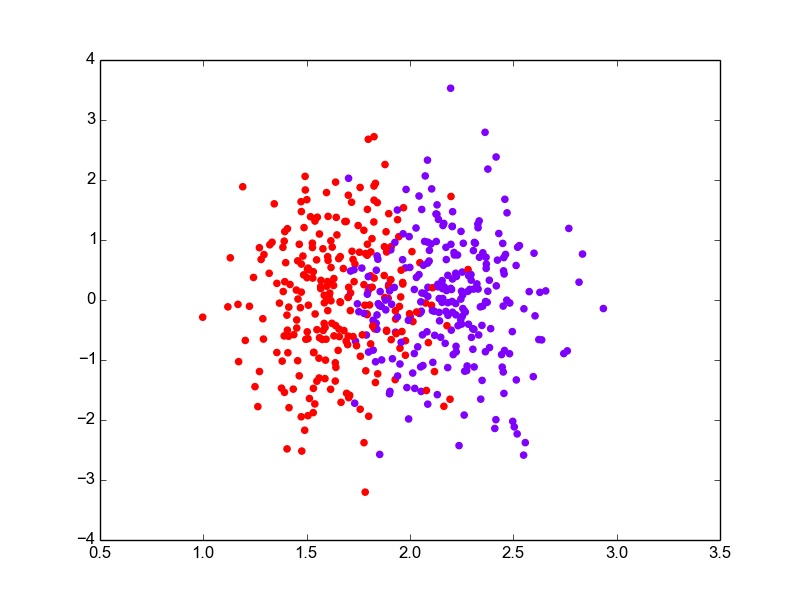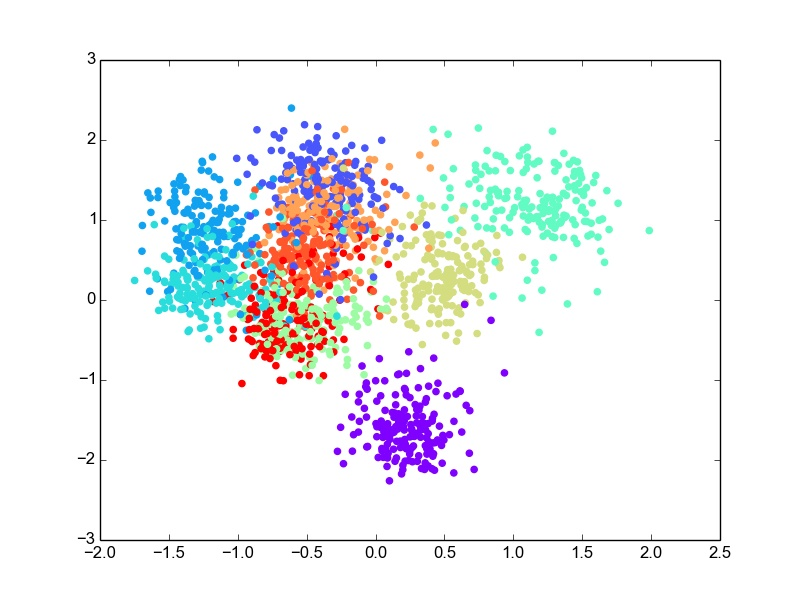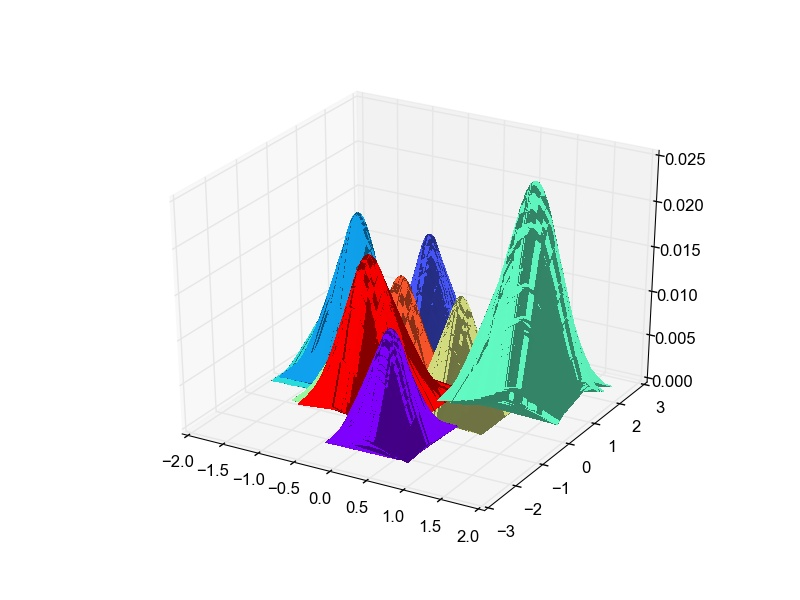# Implementing Fisher’s LDA from scratch in Python

Fisher’s Linear Discriminant Analysis (LDA) is a dimension reduction technique that can be used for classification as well. In this blog post, we will learn more about Fisher’s LDA and implement it from scratch in Python.

### What?

As mentioned above, Fisher’s LDA is a dimension reduction technique. Such techniques can primarily be used to reduce the dimensionality for high-dimensional data. People do this for multiple reasons - dimension reduction as feature extraction, dimension reduction for classification or for data visualizaiton.

### How?

Since this is the theory section, key takeaways from it are as follows (in case, you do not want to spend time on it)
1. Calculate $$S_{b}$$, $$S_{w}$$ and $$d^{\prime}$$ largest eigenvalues of $$S_{w}^{-1}S_{b}$$.
2. Can project to a maximum of $$K - 1$$ dimensions.

The core idea is to learn a set of parameters $$w \in \mathbb{R}^{d \times d^{\prime}}$$, that are used to project the given data $$x \in \mathbb{R}^{d}$$ to a smaller dimension $$d^{\prime}$$. The figure below (Bishop, 2006) shows an illustration. The original data is in 2 dimensions, $$d = 2$$ and we want to project it to 1 dimension, $$d = 1$$.LDA example

If we project the 2-D data points onto a line (1-D), out of all such lines, our goal is to find the one which maximizes the distance between the means of the 2 classes, after projection. If we could do that, we could achieve a good separation between the classes in 1-D. This is illustrated in the figure on the left and can be captured in the idea of maximizing the "between class covariance". However, as we can see that this causes a lot of overlap between the projected classes. We want to minimize this overlap as well. To handle this, Fisher’s LDA tries to minimize the "within-class covariance" of each class. Minimizing this covariance leads us to the projection in the figure on the right hand side, which has minimal overlap. Formalizing this, we can represent the objective as follows.

$$J(w) = \frac{w^{\mathsf{T}}S_{b}w}{w^{\mathsf{T}}S_{w}w}$$

where $$S_{b} \in \mathbb{R}^{d \times d}$$ and $$S_{w} \in \mathbb{R}^{d \times d}$$ are the between-class and within-class covariance matrices, respectively. They are calculated as

$$S_{b} = \sum_{k = 1}^K (m_{k} - m)N_{k}(m_{k} - m)^{\mathsf{T}}$$

$$S_{w} = \sum_{k = 1}^K \sum_{n = 1}^{N_{k}} (X_{nk} - m_{k})(X_{nk} - m_{k})^{\mathsf{T}}$$

where $$X_{nk}$$ is the $$n$$th data example in the $$k$$th class, $$N_{k}$$ is the number of examples in class $$k$$, $$m$$ is the overall mean of the entire data and $$m_{k}$$ is the mean of the $$k$$th class. Now using Lagrangian dual and the KKT conditions, the problem of maximizing $$J$$ can be transformed into the solution

$$S_{w}^{-1}S_{b}w = \lambda w$$

which is an eigenvalue problem for the matrix $$S_{w}^{-1}S_{b}$$. Thus our final solution for $$w$$ will be the eigenvectors of the above equation, corresponding to the largest eigenvalues. For reduction to $$d^{\prime}$$ dimensions, we take the $$d^{\prime}$$ largest eigenvalues as they will contain the most information. Also, note that if we have $$K$$ classes, the maximum value of $$d^{\prime}$$ can be $$K - 1$$. That is, we cannot project $$K$$ class data to a dimension greater than $$K - 1$$. (Of course, $$d^{\prime}$$ cannot be greater than the original data dimension $$d$$). This is because of the following reason. Note that the between-class scatter matrix, $$S_{b}$$ was a sum of $$K$$ matrices, each of which is of rank 1, being an outer product of two vectors. Also, because the overall mean and the individual class means are related, only ($$K - 1$$) of these $$K$$ matrices are independent. Thus $$S_{b}$$ has a maximum rank of $$K - 1$$ and hence there are only $$K - 1$$ non-zero eigenvalues. Thus we are unable to project the data to more than $$K - 1$$ dimensions.

### Code

The main part of the code is shown below. If you are looking for the entire code with data preprocessing, train-test split etc., find it here.

The code is pretty self-explanatory if you followed the theory above and read the comments in the code. Once we estimate the parameters, there are two ways to classify it.

• Thresholding
In one-dimensional projections, we find a threshold $$w_{0}$$, which can be basically the mean of the projected means in the case of 2-class classification. Points above this threshold go into one class, while the ones below go in to the other class. Here is the code to do that.

• Gaussian Modeling
In this method, we project the data points into the $$d^{\prime}$$ dimension, and then model a multi-variate Gaussian distribution for each class’ likelihood distribution $$P(x | C_{k})$$. This is done by calculating the means and covariances of the data point projections. The priors $$P(C_{k})$$ are estimated as well using the given data by calculating $$\frac{N_{k}}{N}$$ for each class $$k$$. Using these, we can calculate the posterior $$P(C_{k}|x)$$ and then the data points can be classified to the class with the highest probability value. These ideas are solidified in the code below.

• Experiments
I used two datasets - Boston housing data and the Digits data.
• Boston data has 13 dimensional data points with continuous valued targets, however one can convert the data into categorical data for classification experiments as well. In this case, I converted it into 2 class data, based on the median value of the target. For this dataset, I explore the threshold classification method. After using the above code for estimating the parameters and a threshold for classification, I evaluate it on the test set, which gives an error rate of ~14%. The 1-D data projections can be plotted to visualize the separation better. I have used the code below.

I have added some random noise to the y-value of the projections so that the points do not overlap, making it difficult to see. Plotting the projections for the entire data, the figure looks something like this.LDA : 1-D Projections for Boston data with added y-noise

• Digits is a dataset of handwritten digits 0 - 9. It has 64 dimensional data with 10 classes. I used this data as it was for classification. For this dataset, I perform the projection of data into 2 dimensions and then use bivariate Gaussian modeling for classification. After evaluation, the error rate comes out to be ~30% on the test set, which is not bad considering the 64-dimesional, 10-class data is projected to 2-D. For the 2-dimensional scatter plot for the data projections looks like this.LDA : 2-D Projections for Digits data

Though there is overlap in the data in 2-D, some classes are well separated as well. The overlap is expected due to the very-low dimensional projection. I think given this constraint, LDA does a good job at projection. I thought it would be fun to plot the likelihood gaussian curves as well. So here is the code to do that and the plot obtained.LDA : Projected data likelihood Gaussian plots for Digits data

Hope this was fun and helpful for you to implement your own version of Fisher’s LDA. If you would like to run the code and produce the results for yourself, follow the github link to find the runnable code along with the two datasets - Boston and Digits.

### References:

1. Bishop, C. M. (2006). Pattern recognition. Machine Learning, 128.